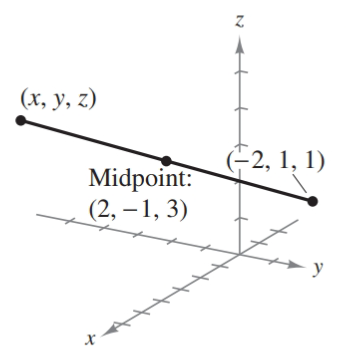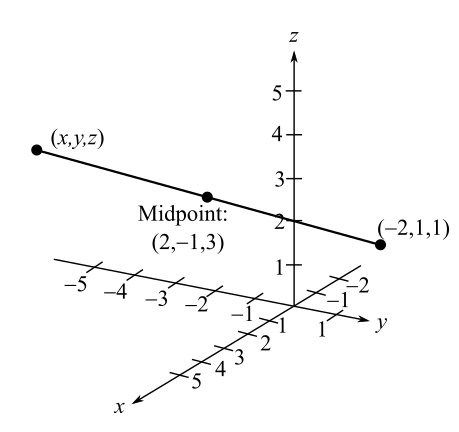Chapter 7.1, Problem 19E### Calculus: An Applied Approach (Min...

10th Edition
Ron Larson
ISBN: 9781305860919

#### Solutions

Chapter
Section### Calculus: An Applied Approach (Min...

10th Edition
Ron Larson
ISBN: 9781305860919
Textbook Problem
5 views

# Using the Midpoint Formula in Space In Exercises 19-22, find (x, y, z).To determine

To calculate: The endpoint coordinate (x,y,z) from the provided figure.Explanation

Given Information:

The provided figure is,

Formula used:

Midpoint formula in space, the midpoint of the line segment joining the points (x1,y1,z1) and (x2,y2,z2).

Midpoint=(x1+x22,y1+y22,z1+z22)

Calculation:

From the provided figure, the midpoint of the line segment is (2,1,3). The endpoint of the line segment is (2,1,1) and (x,y,z)

By the use of midpoint formula in space,

Midpoint=(x1+x22,y1+y22,z1+z22)(2,1,3)=(x+(2)

### Still sussing out bartleby?

Check out a sample textbook solution.

See a sample solution

#### The Solution to Your Study Problems

Bartleby provides explanations to thousands of textbook problems written by our experts, many with advanced degrees!

Get Started

#### In Exercises 914, evaluate the expression. 9. (94)3/2

Applied Calculus for the Managerial, Life, and Social Sciences: A Brief Approach

#### In problems 15-26, evaluate each expression. 25.

Mathematical Applications for the Management, Life, and Social Sciences

#### Evaluate the integral: x3x(x2+3y2)dy

Calculus: Early Transcendental Functions (MindTap Course List)

#### True or False: If a number p is in the interval of convergence of then so is the number .

Study Guide for Stewart's Single Variable Calculus: Early Transcendentals, 8th